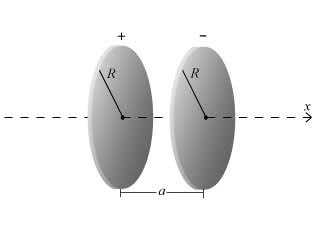# Finite Sheets of Charge Compared to Infinite Sheets of Charge

## Homework StatementConsider two thin disks, of negligible thickness, of radius R oriented perpendicular to the x axis such that the x axis runs through the center of each disk. The disk centered at x=0 has positive charge density n, and the disk centered at x=a has negative charge density -n, where the charge density is charge per unit area.

For what value of the ratio R/a of plate radius to separation between the plates does the electric field at the point x=a/2 on the x axis differ by 1 percent from the result n/epsilon_0 for infinite sheets?

## Homework Equations

E(disk_x) = n/(2epsilon_0) * (1-((x)/(sqrt(x^2 + R^2))))

## The Attempt at a Solution

I have found the electric field at x=a/2 to be:

E(disk_x) = 2*(n/(2*epsilon_0) * (1-((.5a)/(sqrt((.5a)^2 + R^2))))

However, I'm not sure where to go from here. Should I work the above equation to get what R/a is equal to and then set it equal to .99*(n/epsilon_0)?

## Answers and Replies

Doc Al
Mentor
I have found the electric field at x=a/2 to be:

E(disk_x) = 2*(n/(2*epsilon_0) * (1-((.5a)/(sqrt((.5a)^2 + R^2))))
Set that expression equal to .99*(n/epsilon_0) and solve for R/a. (Hint: First rewrite that expression in terms of R/a.)

So now I have:

Sorry this is gonna be messy...

R/a = sqrt(.25*((1-((epsilon_0*E)/n))^-2)-.25) = .99(n/(epsilon_0))

How do I deal with the E? If I sub in the other equation won't that just negate everything? I'm also asked to give the answer to two significant digits, but I don't see how the R/a ratio is going to give me numbers without that E still in it.

Help me out Doc please :)

Okay I got it on my own.

Using the %difference = (E_infinite - E)/(E_infinite)

Since E = n/epsilon_0 * (1-((.5a)/(sqrt((.5a)^2 + R^2)))) approaces n/epsilon_0 as R/a approaches infinity you can plug in n/epsilon_0 for E_infinite and the original equation for E. Solving this for %difference = .01 you arrive at R/a = 50.

Nasty little problem...